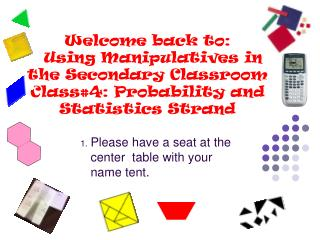DownloadDownload PresentationPlease have a seat at the center table with your name tent.

# Please have a seat at the center table with your name tent.

Télécharger la présentation## Please have a seat at the center table with your name tent.

- - - - - - - - - - - - - - - - - - - - - - - - - - - E N D - - - - - - - - - - - - - - - - - - - - - - - - - - -
##### Presentation Transcript

1. Welcome back to: Using Manipulatives in the Secondary ClassroomClass#4: Probability and Statistics Strand Please have a seat at the center table with your name tent.

2. Class 1: Review Show =1 =1/2 =1/4 =1/3 =1/6 =1/12

3. =1 =1/2 =1/4 =1/8 =1/3 =1/6 =1/12 =1/24

4. =1 =1/2 =1/4 =1/8 =1/3 =1/6 =1/12 =1/24

5. =1 =1/2 =1/4 =1/8 =1/3 =1/6 =1/12 =1/24

6. Area of triangles Determine the area of this triangle as many ways as you can--- discuss How efficient was your approach? Would you approach it differently now?

7. Area of triangles Determine the area of this triangle. Does your method work for this triangle too?

8. Area of quadrilaterals Determine the area of this polygon. Does your method from the triangle work for this polygon?

9. Area of quadrilaterals Determine the area of this polygon. Does your method from the triangle work for this polygon?

10. Class 4: Strand 5 and 6: Probability and Statistics Handouts • Standard 5 and 6 SC 6-8 • Algebra CLG’s – Goal 3 Graphing Calculator and Geoboard